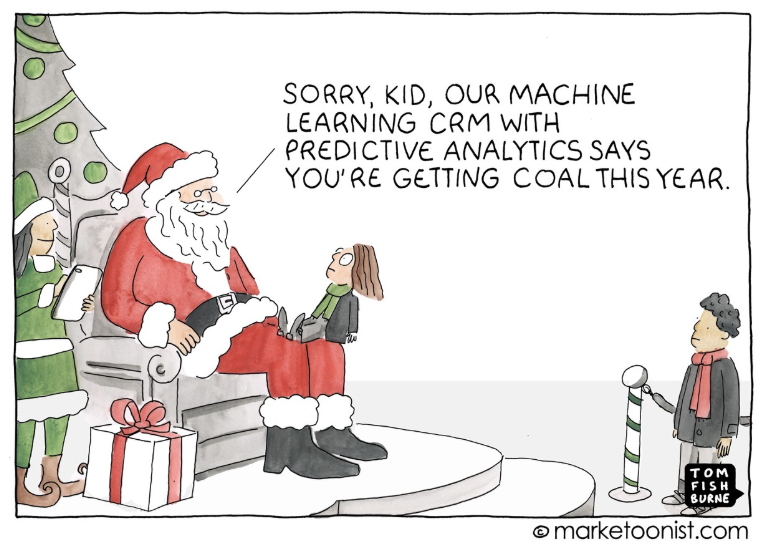# Statistical Modeling — The Full Pragmatic Guide

Source: Deep Learning on Medium# Statistical Modeling — The Full Pragmatic Guide

Interpreting Machine Learning Models — Part 2

Continuing Our series of posts on how to interpret Machine Learning algorithms and predictions.

Part 0 (optional) — What is Data Science and the Data Scientist
Part 1 — Introduction to Interpretability
Part 1.5 (optional) — A Brief History of Statistics (May be useful to understand this post)
Part 2 — (this post) Interpreting models of high bias and low variance. Linear Regressions.
Part 3 — Interpreting low bias and high variance models.
Part 4 — Is it possible to resolve the trade-off between bias and variance?
Part 5 — Local Methods of Interpretability.
Part 6 — Global Methods of Interpretability.

In this post we will focus on the interpretation of high bias and low variance models, as we explained in the previous post, these algorithms are the easiest to interpret so assume several prerequisites in the data. Let’s choose the linear regressions to represent this group of algorithms. If you have no idea what Linear Models are, you might want to check out the article A Brief History of Statistics.

All codes for this post are available on Kaggle.

The purpose here is not to explain what these linear models are or how they work, but how to interpret their parameters and estimates, but a brief introduction may be helpful. Linear models can be simple regressions like OLS, can be regular regressions like Lasso and Ridge,
They can be models for classification such as Logistic Regressions and even for time series such as ARIMA filters. They all have in common the fact that they have linear parameters, that is, when we estimate the “weights” of the variables they are constant for any level. Interestingly, a neural network can also be a linear model if its activation layers are linear (f (x) = x), and such a one-layer network will be similar to our simple linear regression that we will use here, but incredibly less efficient.

Let’s create a theoretical world where we are interested in interpreting the effects of various variables on people’s earnings. In our hypothetical world we have a minimum wage of \$ 1000 and each year of education increases on average \$ 500 in monthly salary. Because our world is stochastic (not deterministic), we have randomness.

When running a regression model, we get the line that produces the smallest possible error: yhat = x * 496 + 1084. That is, the model was able to “understand” the reality we created and estimated that the slope coefficient is ~ 496 (very around \$ 500 that was created) and the ~ 1084 intercept and the interpretation in this case is quite straightforward. He identified the minimum wage (when education equals zero) and when a year of education alters people’s income, \$ 500.

But this case is very simple and very far from reality. By the way, this case is very similar to the model created by Galton in the nineteenth century, the correlation coefficient ‘r’ is the same as R² (only squared). In the real world we have many variables that explain wages, so let’s insert more variables into this model.

In our “world v2” we will have the following behavior, more like reality:

Salaries are explained by three components as follows:
– Grit = Random variable ranging from 0 to 16.
– Education = Random variable from 0 to 16 + part of effort as effort affects how much you educate yourself.
– Experiment = random variable from 0 to 25.

\$ = Grit* 200 + experience* 200 + education* 300 + random part

One way to look at these relationships between variables is through a correlation chart in a heatmap: